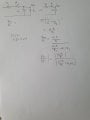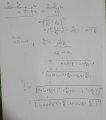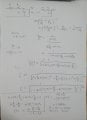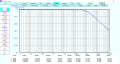# Finding frequency of the filter circuit from the transfer function

#### Wompalamba

Joined Dec 28, 2020
19
Hi i am trying to extract the cutoff frequency from the transfer function that i derived similar to this derivation of the RC filter. I am currently stuck at the step where the equation is modulus on both side as i am not sure how do i continue from there. I have attached the work i have done so far in the image. Thank you for your time!

Edit: i realized that i posted the title wrongly it should be Finding frequency of the filter circuit from the transfer function and i am not sure how to edit the titleLast edited:

#### ZCochran98

Joined Jul 24, 2018
273
Before I answer your question, I do need to point out a small mistake. You have:
$Z = \left(\frac{1}{\frac{1}{sC_1}+\frac{1}{R_3}}\right)^{-1}$
Which is incorrect. You already incorporated the ^-1 with the fraction, so you don't need the ^-1 outside the brackets, and, the sC_1 shouldn't be inverted (1/sC_1 is impedance, so 1/(1/sC_1) = sC_1). So that line should just be:
$Z = \frac{1}{sC_1+\frac{1}{R_3}}$

Now, to answer your question: After you correct that, you have the correct process (Z/Z_total, basically), just the wrong value for Z. Ultimately, after you do all the math, you should get an equation of the form:
$\left|\frac{V_{out}}{V_{in}}\right| = \frac{|a|}{\left|bs+c\right|}$
For some constants a, b, and c which depend on your circuit variables (similar to what you have, but with the corrected value of Z, I can tell you there will be no s in the numerator).

The frequency for which your filter is designed, in this case, is the pole of this equation: what value of s makes the denominator of the fraction = 0? The frequency corresponding to that s (you correctly wrote $$s = \omega j = 2\pi f j$$) is the frequency for which this filter was designed.

Hope this helps!

•Wompalamba

#### Wompalamba

Joined Dec 28, 2020
19
Thank you for correcting my careless mistake and i appreciate you spending some time to share your knowledge. I have since corrected the issue but still have some doubts as to how to solve the modulus function .I am trying to extract out the frequency to find the cut off frequency at $\frac{Vout}{Vin}=\frac{1}{\sqrt{2}}$ when i put in the fixed component values. Am i right to do the following steps as shown in the image attached?#### ZCochran98

Joined Jul 24, 2018
273
You are on the right track. One thing to keep in mind: if you have something = 0, then |something| is also = 0. Thus, at the third line down, where you have written "$$= \frac{1}{1 + sC_1R_1 + \frac{R_1}{R_3} + sC_1R_2 + \frac{R_2}{R_3}}$$," you can actually just go ahead and solve that line, as you set the denominator there = 0, then the magnitude of it will also be zero. Doing that, you can solve for s and then for f, subsequently. The next 3 lines after it are just extra work that, for this case, aren't necessary.

•Wompalamba

#### Wompalamba

Joined Dec 28, 2020
19
Thank you for the guidance. However, I suspect i might have made a mistake somewhere because when i tried to find the frequency cut off it was not the answer i expected which is 810~kHz i have attached the two images below.#### The Electrician

Joined Oct 9, 2007
2,936
At the very end you made an algebra mistake. The expression (C1 R1 - C1 R2) should be (C1 R1 + C1 R2).

•Wompalamba

#### The Electrician

Joined Oct 9, 2007
2,936
Where did you get the value of 810 kHz? I solved it and got the 895.98 kHz value. This is correct for the component values you have given and for the circuit as shown.

•Wompalamba

#### ericgibbs

Joined Jan 29, 2010
18,055
hi W,
This is what LTSpice shows for those component values.
Slight difference to calculated numeric value, due to accuracy of cursor placement.
E

#### Attachments

•Wompalamba

#### ericgibbs

Joined Jan 29, 2010
18,055
hi W,
Did you also try to calculate the Freq, by summing R1 and R2 and say re-naming as R4, in order to simplify the maths.?

Try it.
E

•Wompalamba